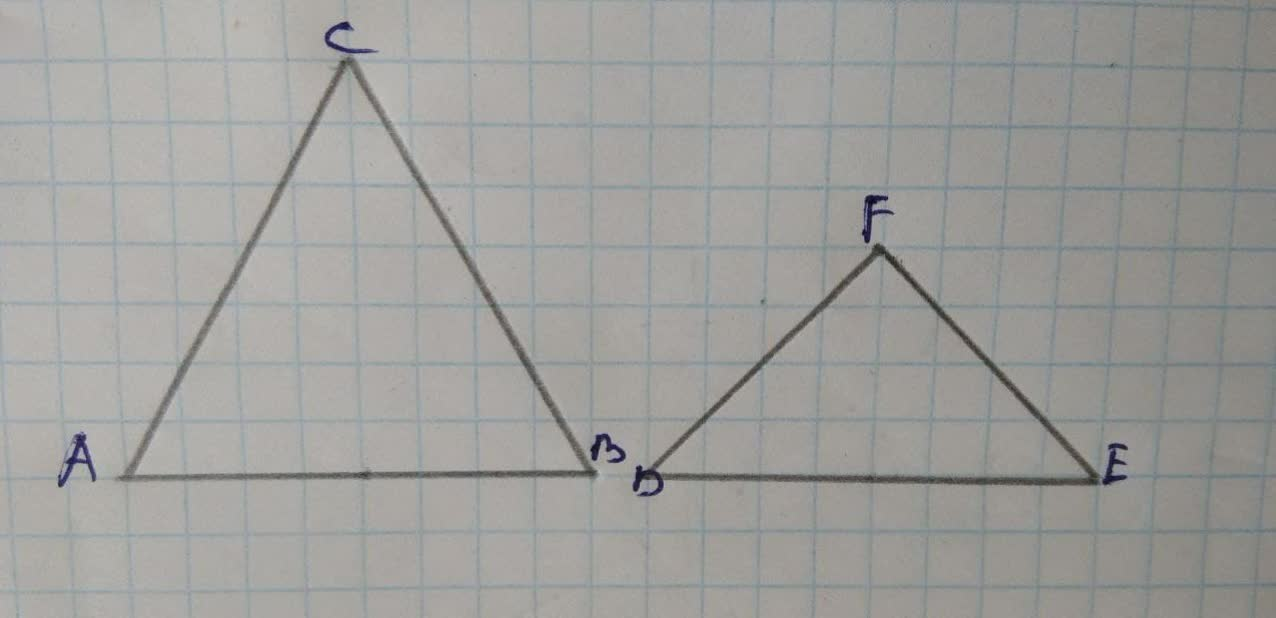## Prove that two isosceles triangles are similar

TrigonometryProve that two isosceles triangles are similar2 years ago
Prove that two isosceles triangles are similar if any angle of one equals the corresponding angle of the other.a. Case 1: Equal Vertex Angles Given: Isosceles△ ABC , isosceles △ DEF, AC = BC, DF = EF, ∠ C=∠ F Prove: △ ABC~ △ DEF Statementc 1.(see above) 2. 3.△ABC ~ △DEF Reasons 1.Given 2. Isosceles triangles with equal vertex angles have equal base angles also.(ex. 2.14 #5) 3. ?